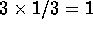Next: Rings Up: Algebraic structures Previous: Algebraic structures

## Monoids and Groups

We need to define a group.

Let us take a set of objects and a rule (called a binary operation) which allows us to combine any two elements of this set. Addition is an example from math, or ANDing in some computer language.

The set must be closed under the operation. That means that when two elements are combined the result must also be in the set. For example the set containing even numbers will always give us an even number when two elements are added together. But if we restrict ourselves to odd numbers, their sum is not an odd number and so we know right off the bat that the set of odd numbers and addition cannot constitute a group. Some books will consider closure in the definition of binary operation, and others add it as one of the requirements for a group along with the ones that follow below.

The set and the operation is called a group if the binary operation satisfies the following criteria:

• the operation is associative, which means it doesn't matter how you group the elements you are operating on, for example in our set of remainders:  + ( + ) = ( + ) + 
• there is an identity element, meaning: one of the elements combined with the others in the set doesn't change them in the least. For example the zero in addition, or the one in multiplication.
• every element has an inverse with respect to that operation. If you combine an element and its inverse you get the identity (of that operation) back.

(Be careful with this last one, -3 is the inverse of 3 in addition, since they give us 0 when added, but 1/3 is the inverse of 3 with respect to multiplication, sincethe identity under multiplication.)

So we can see that the set of natural numbers N (with the operation of addition) is not even a group, since there is no inverse for 5, for example. (In other words there is no natural number which added to 5 will give us zero.) And so the third rule for our operation is violated. But it still has some structure, even if it is not as rich as the ones we'll see later on.

Sets with an associative operation (the first condition above) are called semigroups, and if they also have an identity element (the second condition) then they are called monoids.

Our set of natural numbers under addition is then an example of a monoid, a structure that is not quite a group because it is missing the requirement that every element have an inverse under the operation (Which is why in elementary school 4 - 7 is not allowed.)

What about the set of integers, is it a group?

By itself this question is nonsensical. Why? Well, we have not mentioned under what operation. OK, let us say: the set of integers with addition.

Now, addition is associative, the zero does not change any number when added to it, and for every number n we can add -n and get zero. So it's a group all right.

In fact it is a special kind of group. When we can perform the operation on the two elements in any order (e.g a + b = b + a) then the group is called commutative, or Abelian in honor of Abel. Not every operation is commutative, for example three minus two is certainly not the same as two minus three. Our set of integers under addition is then an Abelian group.Next: Rings Up: Algebraic structures Previous: Algebraic structures

Alex Lopez-Ortiz
Mon Feb 23 16:26:48 EST 1998